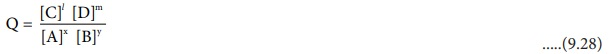Home | | Chemistry 12th Std | Nernst equation

# Nernst equation

Nernst equation is the one which relates the cell potential and the concentration of the species involved in an electrochemical reaction.

Nernst equation

Nernst equation is the one which relates the cell potential and the concentration of the species involved in an electrochemical reaction. Let us consider an electrochemical cell for which the overall redox reaction is,

xA + yB Ōåö lC + mD

The reaction quotient Q for the above reaction is given belowŌłåG = ŌłåGŌĆÖ + RT lnQ                 .....(9.29)

The Gibbs free energy can be related to the cell emf as follows [ Ōł┤ equation (9.24) and (9.25) ]

ŌłåG = - nFEcell; ŌłåGo= - nFEcello

Substitute these values and Q from (9.28) in the equation (9.29)(9.29) ŌćÆ  - nFEcell = - nFEocell + RT ln { [C]l[D]m / [A]x[B]y }       .....(9.30)

Divide the whole equation (9.30) by (-nF)The above equation (9.31) is called the Nernst equation

At 25┬║ C (298K), the above equation (9.31) becomes,Let us calculate the emf of the following cell at 25oC using Nernst equation.

Cu (s) | Cu2+ (0.25 aq, M) | | Fe3+ (0.005 aq M) | Fe2+ (0.1 aq M) | Pt (s)

Given : ( E┬║ )Fe3+|Fe2+ = 0.77V and ( E┬║ ) Cu2+ | Cu = 0.34 V

Half reactions are

Cu (s) ŌåÆ Cu2+ (aq) + 2e-                        ..... (1)

2 Fe3+ ŌåÆ (aq) + 2e- ŌåÆ 2Fe2+ (aq)              ..... (2)

the overall reaction is

Cu (s) + 2 Fe3+ (aq) ŌåÆ Cu2+ (aq) + 2 Fe2+ (aq), and n = 2

Apply Nernst equation at . 25┬║C .Given standard reduction potential of Cu2+ | Cu is 0.34V

(Eoox )Cu|Cu2+ = - 0.34V

(Eored) Fe3+|Fe 2+ = 0.77V

E┬║cell = - 0.34 + 0.77

E┬║cell = 0.43V### Evaluate yourself

The electrochemical cell reaction of the Daniel cell is

Zn (s) + Cu2+ (aq) ŌåÆ Zn2+ (aq)+Cu (s)

What is the change in the cell voltage on increasing the ion concentration in the anode compartment by a factor 10?

Tags : Thermodynamics of cell reactions | Electro Chemistry , 12th Chemistry : UNIT 9 : Electro Chemistry
Study Material, Lecturing Notes, Assignment, Reference, Wiki description explanation, brief detail
12th Chemistry : UNIT 9 : Electro Chemistry : Nernst equation | Thermodynamics of cell reactions | Electro Chemistry